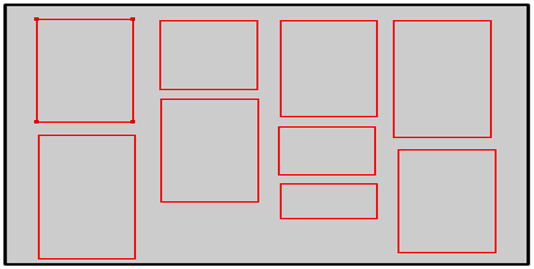# 前端页面–瀑布流布局的实现#main{
position: relative; text-align: center; margin: 0 auto;
}


margin 简写属性在一个声明中设置所有外边距属性。该属性可以有 1 到 4 个值。这个简写属性设置一个元素所有外边距的宽度，或者设置各边上外边距的宽度。在这里要注意一下的是在css中，margin和padding这样的属性设置值的时候都是顺时针设置的，也就是上，右，下，左，这个顺序来的。

//动态添加瀑布图片的功能函数
function waterfall() {
//取得展示框对象
var $boxs =$("#main>div");
// 一个块框的宽
var w = $boxs.eq(0).outerWidth(); //每行中能容纳的展示框个数【窗口宽度除以一个块框宽度】 var cols = Math.floor(($(window).width() - 30) / w);
//给最外围的main元素设置宽度和外边距
$('#main').width(w * cols).css('margin', 'o auto'); //用于存储 每列中的所有块框相加的高度。 var hArr = [];$boxs.each(function(index, value) {
var h = $boxs.eq(index).outerHeight(); if (index < cols) { hArr[index] = h; //第一行中的num个块框 先添加进数组HArr } else { var minH = Math.min.apply(null, hArr); //数组HArr中的最小值minH var minHIndex =$.inArray(minH, hArr);
$(value).css({ 'position': 'absolute', 'top': minH + 'px', 'left': minHIndex * w + 'px' }); //数组 最小高元素的高 + 添加上的展示框[i]块框高 hArr[minHIndex] +=$boxs.eq(index).outerHeight(); //更新添加了块框后的列高
}
});
}


//监听滚动条
window.onscroll = function() {
if (checkscrollside()) {
var $lastBox =$('#main>div').last();
var lastBoxDis = $lastBox.offset().top + Math.floor($lastBox.outerHeight() / 2);
var scrollTop = $(window).scrollTop(); var documentH =$(window).height();